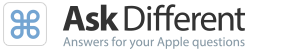## How Unsung am I?

0

(click on this box to dismiss)

Zero and non-zero accepted count. Self-accepted answers do not count.Q&A for power users of Apple hardware and software

``````-- How Unsung am I?
-- Zero and non-zero accepted count. Self-accepted answers do not count.
DECLARE @MAXDATE DATETIME

SELECT @MAXDATE = MAX(Date)

with newbies as
(
select top 100 * from badges where
name = 'Unsung Hero'
order by date desc
),
(
select max(date) as date from newbies
)
select
sum(case when a.Score = 0 then 0 else 1 end) as [Scored Answers],
sum(case when a.Score = 0 then 1 else 0 end) as [Unscored Answers],
sum(CASE WHEN a.Score = 0 then 1 else 0 end)*1000 / count(a.Id) / 10.0 as [Percentage Unscored],
max(datediff(d, n.date, @MAXDATE)) as dayspassed
from
Posts q
inner join
Posts aHold tight while we fetch your results